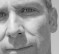Started by 7 years ago2 replieslatest reply 7 years ago1554 views

Hi all, i have some problems with my msp432 (#MSP430) and adc14.

I want to have a sample every 5 microseconds. So i have set smclk = 1Mhz (dco = 24Mhz and then divided) and ta0ccr0 = 5 so that every interrupt i should have a sample. Furthermore i have set adc14 clock = smclk (ADC14SSEL_4) and clock cycles (32 cycles sh + 16 cycles conversion --> 32+16 / 12 = 4 < 5us).

Is correct this reasoning? I post a part of my code:

Thanks for help.

Luca

// ==========Configuration clock 24Mhz - Smclk 12Mhz==========
CSKEY = 0x695A;                    // Unlock CS module for register access
CSCTL0 = 0;                        // Reset tuning parameters
CSCTL0 = DCORSEL_4;                // Set DCO to 24MHz
CSCTL1 = CSCTL1 & ~(SELS_M | DIVS_M); // Clear existing registers
CSCTL1 = CSCTL1 | (SELS_3 | DIVS_1); // For SMCLK = 12Mhz, select DCO as source then divide by 2
CSKEY = 0;                         // Lock CS module from unintended accesses

// ==========Configurazione ADC 14 bit (24Mhz)==========
P6SEL1 |= BIT1
P6SEL0 |= BIT1;    // p1.6 ad
<span></span>ADC14CTL1 = ADC14RES__14BIT;                      // Use sampling timer, 14-bit conversion results (16 clock cycles)

// ==========Configurazione TimerA0==========
NVIC_ISER0 = 1 << ((INT_TA0_0 - 16) & 31);  // Enable TA0_0 interrupt in NVIC module
TA0CCTL0 &= ~CCIFG;                         // Interrupt disable
TA0CCR0 = 5;                                // Overflow (step dell'interrupt)
TA0CTL = TASSEL__SMCLK | MC__UP | ID_2;     // SMCLK, UP mode, division 4 (12 MHz / 4 = 3 MHz)
TA0EX0 |= TAIDEX_2;                         // division 3 (3 MHz / 3 = 1 MHz)

// ==========Timer A0 interrupt service routin==========
void TimerA0_0IsrHandler(void) {
TA0CCTL0 &= ~CCIFG;
// I want adc sampling only in this period (0-1000). Every 5us i have time to have sample
if(microsecondi > 0 && microsecondi <= 1000) {
index_N++;
}
microsecondi += STEP;
// There is a rtc that repeat this cycle (this works)
if (microsecondi >= 10000){
microsecondi = 0;
}

[ - ]Hey Luca, if you tell a little more about what you mean by 'some problems', you stand more chances to receive help from other members.

[ - ]